### Diary

Belfry Bulletin## A Brief Review of the Theory Available to the Cave Surveyor

By Mike Luckwill

### Introduction

Clarke Plane and Geodetic Surveying for Engineers.  London: Constable, 1963. 2 vols.

Allan, Hollway and Maynes.  Practical field surveying and computations.  London: Heinemann 1968.

Rainsford.  Survey adjustment and least Squares.  London.  Constable, 1957.

Many surveyors worship the tin-god called the close traverse by means of a ritual called closing the traverse.  Unfortunately they seem to have forgotten the basis of this dogma, and, sadly, seem unable to apply it in the more complex cases when it is of more value.  It does seem important the hours of hard, dedicated caving required to obtain survey data, the maximum amount of information is not always extracted; or, even worse, more then the maximum is magically produced from nowhere.

In order to put the procedures into some kind of context we shall imagine ourselves faced with surveying a complex cave to a high degree of accuracy (at least C.R.G. grade 6).  The resulting survey will be a complex network in three dimensions.  The surveyed line between two nodes on this network will be called a traverse and is fully defined by three numbers and a datum point, such as one node.  In order to simplify the rest of the paper, each of these three numbers will be loosely referred to as a distance, elevation or bearing.  Also the reader should remember that any calculation using distance will in practice be triplicated.  The aim of the field work is to measure all the traverses; this work is planned by means of theoretical calculations beforehand and is followed by computations based on more theory.

### Errors

Wherever a measurement is made it is accompanied by an error.  Errors are of three types; gross, systematic and random.  Gross errors are mistakes on the part of the surveyor and they must not occur; although this is easy to say, it is not always easy to practise, but as it really a practical matter and not theoretical one shall leave it. Systematic errors are difficult to detect in the field; every precaution should be taken to either remove them by careful calibration or turn them into random errors.  Random errors cannot be avoided but are practicable and amenable to statistical analysis.  We shall assume that in every case random errors are normally distributed although this is not in fact the case for indirectly found quantities.

### Statistics

If a line AB is surveyed n times, the results will be distributed about a mean, as in Fig.1. If each result has an associated error e, the error associated with the mean will be e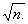hence the usefulness of making a measurement as many times as possible. The mean is called the most probable value (m.p.v.). The difference between the mean and the true value is called the systematic error and one of the aims of the surveyor is to reduce this as much as possible.  Since the true value can never be found (if it were it would never be recognised as such) the object of the survey is to find the m.p.v.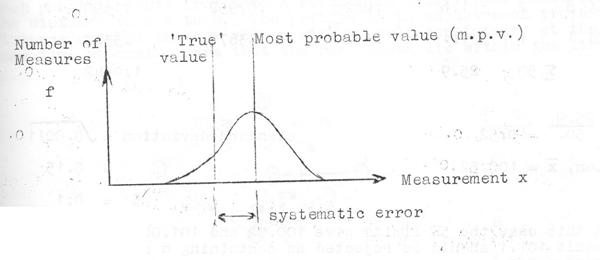FIGURE 1.

From a knowledge of the mean,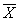, the standard deviation,, can be obtained:The standard deviation is a measure of the spread of the results about the mean and so represented the precision of the set of results.  It is very important to note that it is possible to have a precise set of results and a high systematic error, i.e. high precision and low accuracy.

The badly named probable error, E is related to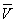by E=0.6745. Less than 1 result in 1000 will lie outside the limits +3E and -3E.  any result lying outside the limits 5E and -5E can safely be rejected as being incorrect. An example is now worked.

Example 1.

Calculate the mean and standard deviation of the following set of results.  Should the value 101.1 be rejected?

Using an assumed mean of 100:

 X f xf x -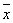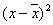f100.2 1 0.2 -.318 .1011 .1011 100.3 6 1.8 -.218 .04752 .2851 100.4 10 4.0 -.118 .03192 .1392 100.5 13 6.5 -.018 .00324 .0421 100.6 12 7.2 .082 .00674 .0809 100.7 5 3.5 .182 .03312 .1656 100.8 2 1.6 .282 .07952 .1590 101.1 1 1.1 .582 .3387 .3382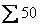25.9 1.0542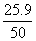= 0.52                                                 standard deviation =mean,= 100.52                                                                                                   =   0.15

E  =   0.1

In this case the 5E limits give 100.02 and 101.02. thus the result 101.1 should be rejected as containing a systematic or gross error.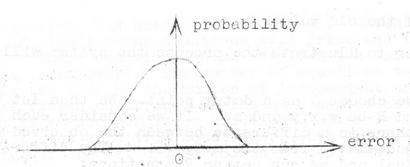FIGURE 2.

The probable error may also be used in planning the survey: if, for example, co-ordinates are required to 1m, the survey should be designed to give a probable error of 0.3m, with the expectation that only one co-ordinated in 1000 will be outside the required precision.

The normal distribution curve also gives the probability of obtaining any result (Fig.2).  The probability that two results will differ by 3is approximately 0.0045.  If two such results are obtained than a systematic error is suspected.  The maximum systematic error that can pass undetected is therefore related to the standard error, which must be known beforehand.  The standard error may either be found by analysing survey data obtained with the instruments, or may be calculated theoretically from a knowledge of the instruments.  In the latter case a check should be made to ensure that these theoretical predictions are being realised in practice.

Having performed the pre-survey calculations, we make the survey and calculate the internode distances. We now have a three dimensional network with all the internode distances known.  If we were to attempt to draw the survey at this stage we should quickly discover that the data is not self-consistent.

A reference to Fig.3 which shows the distances along 7 traverses in a hypothetical system, will show that, for example, each possible route from A to E results in a different value for the distance A to B.  The problem is to adjust each traverse distance do that we, must ensure that the new values lies within the limits of error of the old values.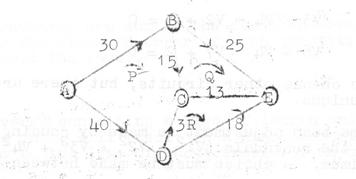FIGURE 3

In order to illustrate the process the system will now be adjusted: -

First we choose A as a datum point.  We then let the m.p.v.s. of B,C,D and E be w,x,y and z.  If we consider each individual traverse, there is a difference between the observed distances and those calculated from the m.p.v.s.  The difference is called a residual and we can set up 7 equations:

Line.

 AB BC BE CE AD DC DE V1 = w  30 V2 = x  w  15 V3 = z  w  25 V4 = z  x  13 V5 = y  40 V6 = x  y  3 V7 = z  y  18

Using the theory of least squares; the minimum amount of disturbance to each value is obtained by making the sum of the squares of the residuals a minimum.  The equation is obtained from one observation (the distance along a traverse).

There is another set of equations which we might use: if we call the m.v.p.s. of the distances AB, BC, BE, CE, AD, DC, DE; d1, d2, d3, d4, d5, d6, d7, then by taking the three circuits P, Q and R we have:

P = d1 + d2  d6  d5 = 0

Q = d3 - d4  d2 = 0

R = d6 + d4 d7 = 0

These are called condition equations, since they refer to the condition that a closed traverse the distances add up to zero.  Putting d1 = V1 + 30, etc., we now have three equations:

V1 + V2  V6  V5  2 = 0

V3  V4  V2 + 3 = 0

V6 + V4  V7  2 = 0

It is possible to choose other circuits, but there are only three which are unique.

Once again the best adjustment is made by causing the sum of the squares of the residuals (v12 + v22 + V32 + V42 + V52 + V62 + v72) a minimum.  A choice must be made between using the observation equations and using the conditions. Whichever is chosen a set of simultaneous equations will arise and it is the solution of these equations which involves the labour (or preferable a computer).  The number of equations to be solved will be the same as the number of independent unknowns if we use observation equations, or the same as the number of conditions if we use condition equations.  Clearly in this example we shall use condition equations. When the three equations involved are set up and solved we find that the residuals are:

 V1 = - 4/24,   V5 = 4/24, V2 = 3/24,   V6 = -25/24, V3 = -35/24,   V7 = 29/24. V4 = 6/24,

The adjusted distances then become: AB = 29BC = 16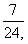BE = 23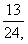AD = 40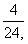DC = 1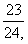DE = 19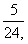CE = 13The reader is left to check that the adjusted distances are self- evident.

Having obtained an adjusted traverse distance, the position of the individual stations along the traverse must now be adjusted.  There is no theoretical basis for either of the methods available do it, it is usual to use the simplest.  The two procedures are that of Bowditch and the Transit method.  As the Transit method does not adjust the bearings as much as Bowditchs method it is to be preferred in the case of a magnetic survey; it involves an adjustment in, the easting of each leg, equal to the mis-closure in the easting multiplied by the easting of the leg and divided by the total eastings of the traverse.  The Bowditch method involves the easting, say, multiplied by the length of the leg and divided by the total length of the traverse.

### Combination Of Errors

We have seen that we must predict the probable error of each traverse: this is obtained by combining the errors associated with each leg.

If n quantities are summed the standard error of the sum is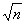times the standard error of each quantity (assuming these to be all the same).

#### Example 11.

PQ is a traverse containing n legs of length and bearings a1.  An error da1 in a1 will result in a displacement of Q at right angles to the line of the traverse of an amount sda1.  If the standard error of these errors is e, then the standard error of the position n of Q will be given by:

E = ±se#### Example 111

PQ is a traverse containing n legs of length s and clockwise included angles a1 with errors as above.  The displacement of Q at right angles to the line of the traverse will be (n  i)sda1, caused by an error in a1.  The standard error of the position of Q will be given by:

E = ±se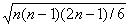A quick comparison of these two results will show why the prismatic compass was considered superior to the astrocompass when accurate cave-surveying first started.  Since the prismatic compass is now being used to the limit of its precision by one or two surveyors, it will soon be replaced by a theodolite; the latter instrument will be more precise, however, than an astrocompass.

Knowing the error, E, produced by the angular errors, we can plan the precision of the taping; which should produce a standard error of not more than E/in each of n legs.

If the traverse is in the form of a loop the easting and northing errors will be about 1/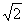of the error of a straight traverse of the same length.

### Expressions Of Accuracy

A C.R.G. grade 7 covers a multitude of sins.  Only one of the many forms in which the accuracy of a survey may be expressed will be discusses: it has the advantage that it enables traverses of different lengths to be compared.  The factor Q is defined by:

Q = t/L½

where t is the total misclosure (true distance) and L is the total length of the traverse.  Note that the factor has units.  A misclosure of 1 metre in 1000 metres gives Q = 0.03. perhaps this is a suitable target for cave-surveying during the next view years!

### Weighting

No mention has been made so far of the ability of the surveyor to weight his data.  Every computation so far discussed can be readily modified to take account of weighted results: the problem is a practical one and of utmost importance.  Data of equal reliability have equal weights.  Rarely will surveyed data or calculated data have equal reliability and so the surveyor has to attach a weight to each piece of data.  For length measurements it is usual to assume that the weights are inversely proportional to the length of the line; however, the conditions involved in cave surveying are so different from surface work that this is no longer a satisfactory assumption.  Since the purpose of this article is not only to review established procedures, we shall not speculate upon the problem.  There is no doubt, however, that the problem must be solved, either by theoretical analysis of survey data, before cave-surveying can be considered an accurate metrology.

### Summary

We have considered the complex case of high accuracy.  The surveyor is at liberty to simplify or omit the procedures available whenever the accuracy of the survey is too low to warrant them.  However, in some cases any attempt to simplify only results in confusion. For example, if a complex system has been surveyed to a low accuracy, it may be just as well to adjust the results using the principle of least squares, knowing that the result will at least appear at the end of the work, as to try to adjust the results by inspection of the drawing which may easily lead to difficult decisions having no theoretical procedures available.

Whatever procedures are used it is most important that as well as giving the results, in the form of co-ordinates perhaps the standard error, or probable error, is given and the size of the misclosure stated.  In this way the user of the survey will not only be able to interpret the data correctly, but will be assured that the surveyor is himself aware of the accuracy of the work.